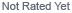Loading... Please wait...

# Understanding Energy and Power

## Understanding Energy and Power

Understanding Energy and Power

Solar panels produce power and energy from sunlight, and the electrical devices that you use every day (like lights, iPods, and washing machines) use power and energy while they’re running. Now, unless you’ve taken a physics class at some point, you may be a little fuzzy on the difference between these two things. So let’s say you want to use a solar panel to run a certain electrical device. The first step in doing this is to understand the difference between power and energy.

The explanation starts with the units. Solar power is measured in a unit called a Watt (W), and solar energy is measured in a unit called a Watt-hour (Wh) or kilo-watt-hour (kWh).

The prefix “kilo” means “1000”, so 1 kWh is the same as 1000 Wh.

As the units for power and energy show, the difference between them is time – in this case, an hour (h). Mathematically, this can be written:

Energy = Power X Time (Equation 1)

In English, what this means is that energy is power happening over time. We could get fancy and show you some more equations, but really, Equation 1 is all you need to know for now.

If you look at an electrical device in your home, like a lightbulb, you will find (somewhere on the device) that it is rated according to the power (W) that it uses while it is running. If you look at your monthly electric bill, you will see that you are being charged by the amount of electrical energy (kWh) that you use in a month.

To get from one to the other, simply use Equation 1: multiply the power (W) that the device is rated at by the time (hours) that it will be running.

So for example, if you want to know how much energy it will take to run a 10W lightbulb for 1 hour, you would calculate:

10 Watts X 1 hour = 10 Watt-hours of energy

If you wanted to run this lightbulb for 5 hours, you would be using:

10 Watts X 5 hours = 50 Watt-hours of energy

If you wanted to run this bulb for 5 hours every day, you would be using 50Wh/day.

Now, if you look at the back of a solar panel, you will see that it is rated according to the power (W) that it produces. So you might think that you could calculate the energy a solar panel could produce in a day by using Equation 1 to multiply the power output of the panel by the number of hours that the sun is out. Unfortunately, there is one small complication: the amount of power that a solar panel produces depends on the amount of light, and that changes throughout the day. A solar panel will only produce its full rated power in what is called a “Peak Sun”.

Fortunately, the National Renewable Energy Laboratory has published solar insolation maps to help work around this complication. In our next article, we’ll talk more about solar panel ratings and insolation maps and how they can be used to calculate the Wh that a solar panel can produce in a day.

## New Products

•••••